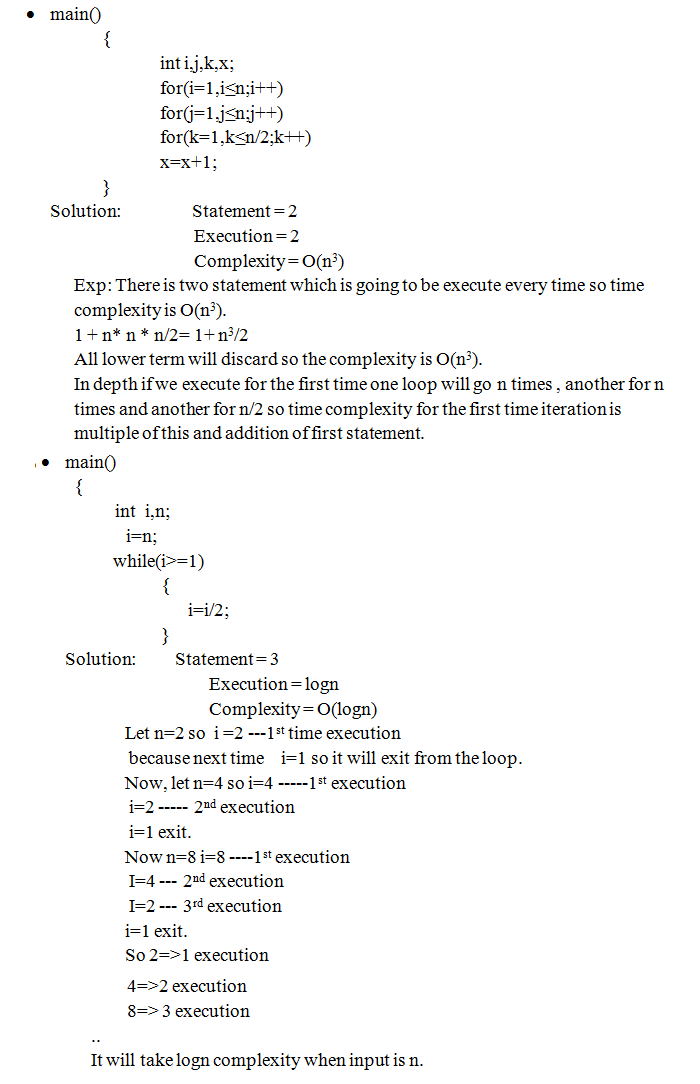## DESIGN AND ANALYSIS OF ALGORITHM

Find out some examples based on Time Complexity
1 November, 2016

main()
{
int i, j, x;
x = x+1;
for(i=1; i<=n;i++)
x = x+1;

for(i=1; i<=n;i++)
for(j=1; j<=n;j++)
x = x+1;
}

Solution:       Statement      =   4
Execution       =   4
Complexity    =   O(n2)
Exp:  There is four statement which is going to be execute every time so time complexity is O(n2).
1 + 1+ n + n2
NOTE: All lower term will discard so the complexity is O(n2) . ALL ARE IN POWER OF n.

main()
{
Int i,n;
i=n;

while(i>1)
{
x=x+1;
i=i/2;
}
}

Solution:       Statement      =   2
Execution        =  2
Complexity     =  O(n3)
Exp:  There is two statement which is going to be execute every time so time complexity is O(n3).
1 + n* n * n/2      =     1+ n3/2

All lower term will discard so the complexity is O(n3).
In depth if we execute for the first time one loop will go n times ,
another for n times and another for n/2 so time complexity for the first
time iteration is multiple of this and addition of first statement.function (int n) {
If(n == 1) return;
for(int i=1 ; i<= n ; i++)
{
for(int j=1; j<=n; j++)
printf(“”);
break
}
}

Solution:
Statement    = 1
Execution     = 1
Complexity   = n
The complexity of the function is O(n).
Even though the inner loop is bounded by n , but due to the break statement it is executing only once.